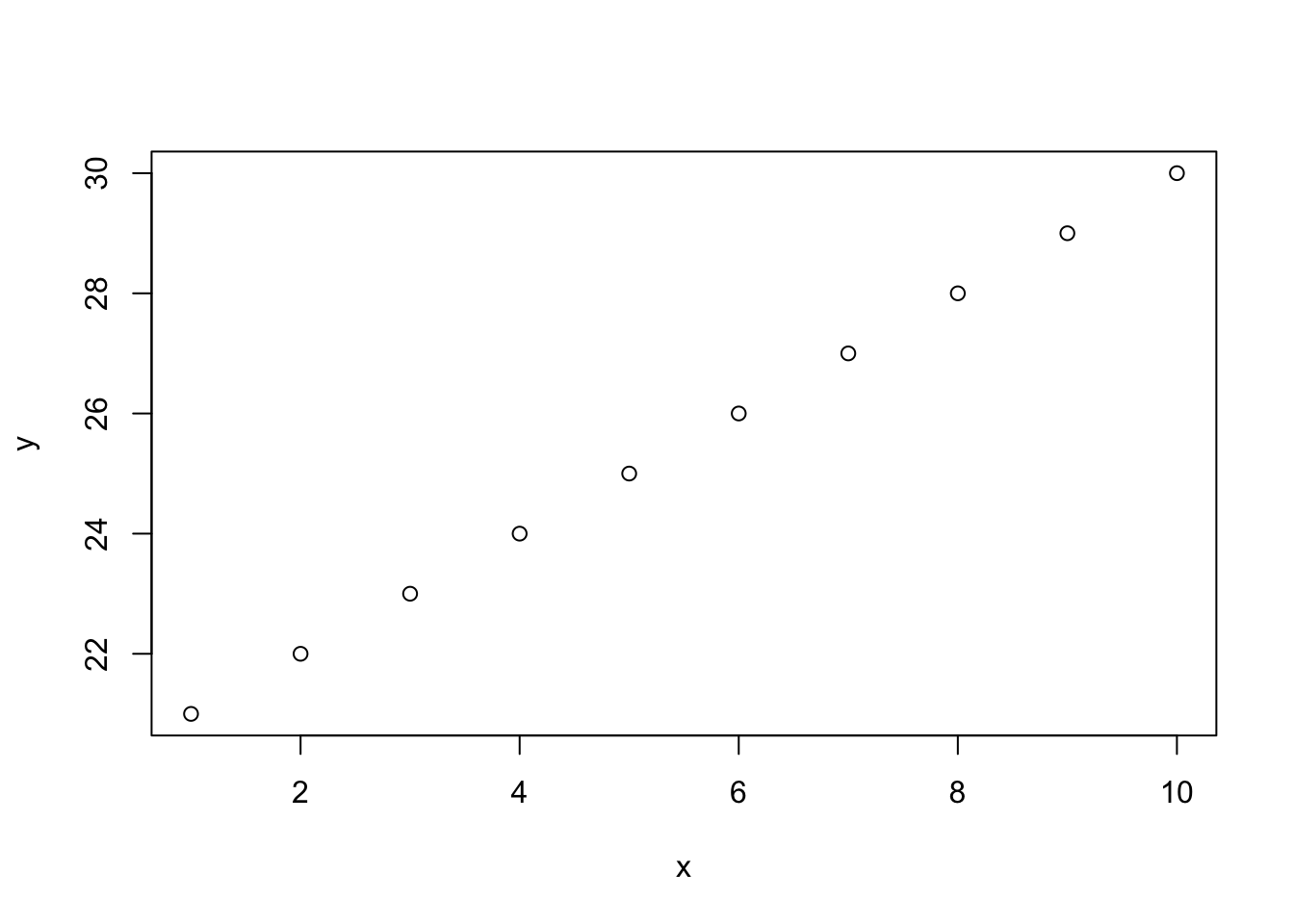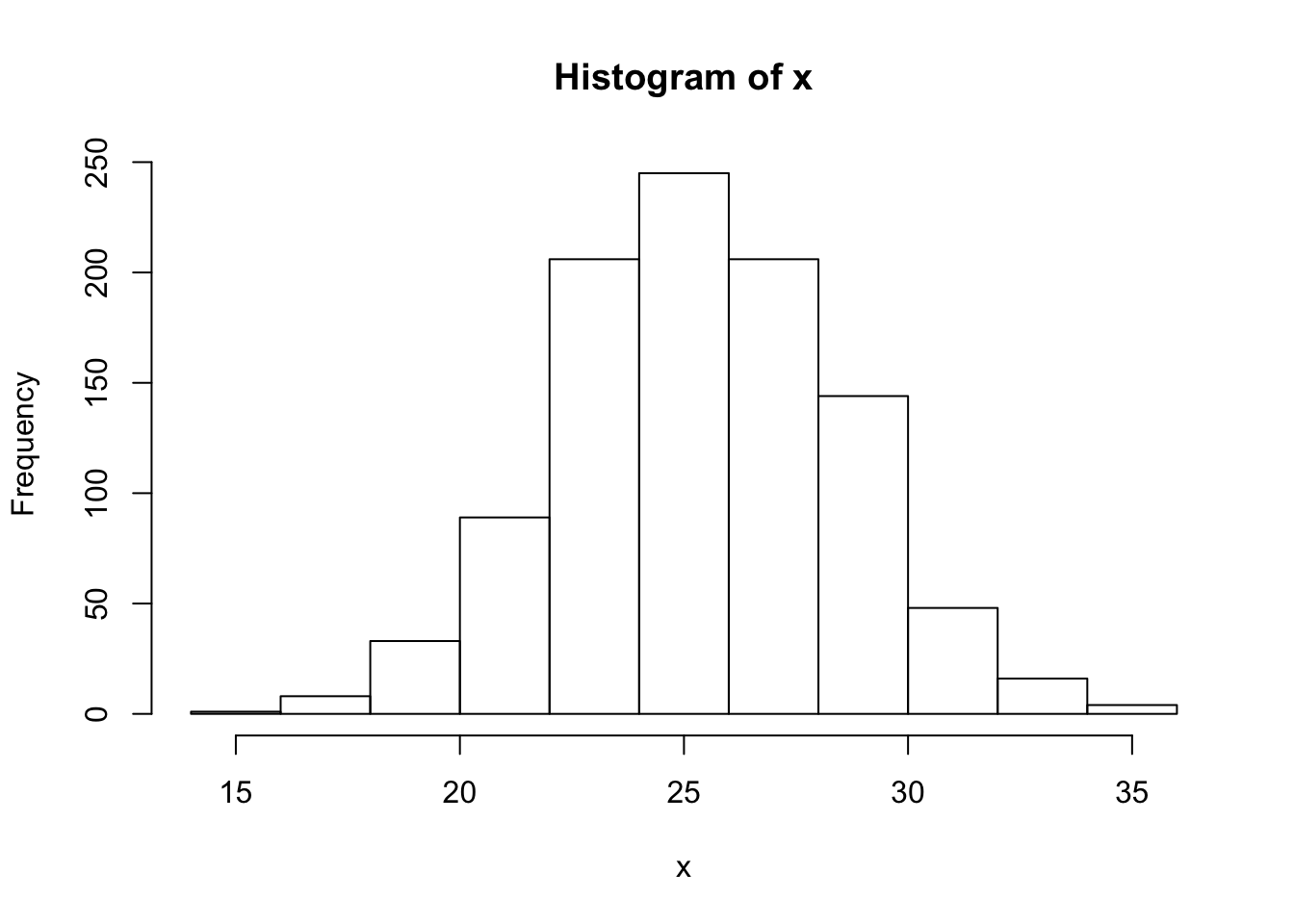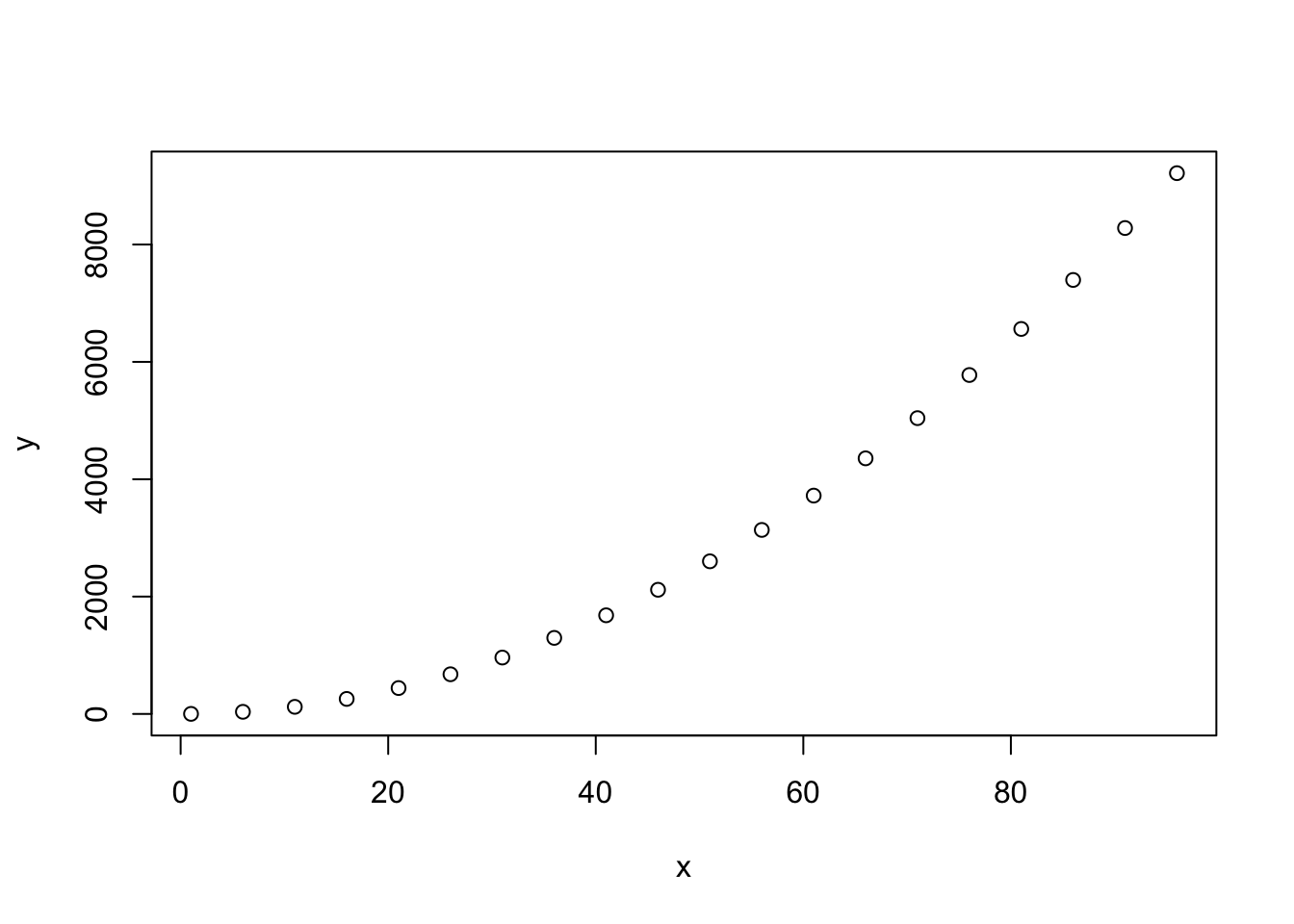What to expect

In this section we are going to:

• learn what R is, and start using R as a tool
• understand how to handle data structures in R
• get familiar with plotting and visualising data
• handle datasets and reading in data

Introduction

For modern evolutionary biologists, handling large amounts of data is a fundamental skill. Familiarity with a programming language, particularly one that makes it straightforward to visualise, explore and filter data is the best way to achieve this ability. There are many different types of programming and scripting languages; the entire concept may seem daunting at first, especially if you have never encountered it before. This is natural, many other biologists applying scripting tools on a daily basis have started from similar first principles. A little patience with the basics of any form of programming and you will soon be able to do much more than you thought possible.

For this section, we will be introducing you to R, a statistical programming language and environment that is widely used in the biological sciences. R is flexible, clear and easy to learn. It also extremely good for producing quick, high quality visualisations of data, which makes it very useful for anyone trying to explore their data. Perhaps the greatest strength of R is its focus on statistics - this makes it an excellent tool for carrying out and learning statistical analysis. R is also used for data analysis beyond evolutionary biology - it forms the basis of data science for companies such as Google, Facebook and Twitter, among many others. If you find yourself wondering why you are learning a programming language, it is worth remembering this point - familiarity with R and scripting will provide you with a very flexible and useful skill.

We believe the best way to get an idea of what R is and what it is capable of is to dive straight in. There really is no better way to learn and understand than to demonstrate the workings of the language to yourself. As a brief overview, we will show the utmost basics here before moving onto more advanced topics in the next chapter. We will also introduce some basic statistical concepts for which R makes visualisation and understanding straightforward. Together these first two chapters will form the foundations for applying R to more evolutionary genetics focused questions.

Getting set up

To begin, you should download and install R from the CRAN. This is the online hub for the R language and it stands for Comprehensive R Archive Network. Be sure to download the correct R installation for your operating system.

We also strongly recommend you install RStudio, a front-end for R. This utility makes working in the R environment a lot more straightforward, standardises things across operating systems and has many helpful features. For the purposes of these tutorials, we will assume you are using RStudio.

With both R and RStudio installed, start Rstudio and we will begin!

Familiarising yourself with the R environment

Now RStudio is loaded, you should see three panels, the console, a panel called environment and one with at least four tabs labelled files, plots, packages and help.

With R, you type commands into the console and then this replies with output. R will operate from within the directory it is started from. This is an important point to remember for later but for now, we will settle with using a single function in order to find out which directory we are in and also get an idea of how this all actually works. In the console, simply type the following:

getwd()

When you type this, you should see the directory that you are in printed in the console. Knowing where R is operating is important for understanding how to read data into the environment, but we will explore this concept more a little later. What is more important to understand is that you typed a function into the R environment, R evaluated it and provided you with an answer. If you want to learn what a function does, you can simply ask R for help. Lets try this with getwd().

?getwd

This should open up a help dialogue. Help pages for functions like this are extremely useful and are very good for getting an idea of what the functions do and how you can use them. There are even examples of how to run them. For a beginner, some of the information in this dialogue will likely seem hard to understand but in time you will be able to read them effectively!

Let’s get used to interacting with the R console. You have already typed a function once and also called for help. You did this at the prompt which should appear like this in the R console.

>

This is called the prompt because it is waiting for your input in order to respond. Try typing a few numbers like below. What output do you see?

1
10+10
50-5
50*2000
9/3
20:30

You will probably have noticed that R echoes single numbers back to you, but it also acts like a calculator and actually processes the numbers you enter into it. Characters such as +, -, / and * are operators that mean add, substract, divide and multiply respectively. Using : tells R to print all numbers between the start and end values.

In addition to basic mathetmatical operations such as multiplication, division, addition and subtraction, you can use R to perform logical operations. For example you can ask whether two values are identical or whether one is greater than the other.

# are the values equal?
2 == 2
# is the first value greater than the second?
5 > 10
# is the first value less than or equal to the second
9 <= 10

When you run this code in the console, R will return TRUE or FALSE - denoting whether the logical statements you made are indeed true or false. The examples here are quite trivial, but this is a powerful feature of R (and indeed programming in general) that forms the basis of creating your own functions and performing more complex operations. As a side note, the lines that start with # are comments in our R code - R will not interpret them. These comments are useful when you are writing scripts (see next section) as a reminder for what your code is doing!

R doesn’t just interpret numeric values, it can also handle character information - i.e. words and text. If you type a word in quotes or double quotes, it will repeat it back to you. Like so:

"Hello world!"

Take note of the fact that if you do the same without quotes, it will not work - you will get an error.

Hello world!

As you might already be thinking, typing in single words or numbers like this isn’t of much value, but as you will come to see, this forms the basis of more powerful ways of storing information.

Variables, vectors and assignment

Now that we have interacted with the console and typed some values in, this is a good time to visit the statistical concept of variables.

Numeric vectors

In terms of evolutionary biology and biostatistics, a variable is any characteristic or measurement that varies among individuals. There are many different types of variable - the first type we will examine is numerical. For example, in R, a set of numerical variables would look like so:

c(10, 50, 10, 40)

Let’s breakdown what we did here. The c function is a basic one that you will use a lot - it combines values into what we call a vector. You can think of a vector as one way of storing a set of variables. Take a closer look at c and see that by separating arguments with a comma, you can combine as many values as you want.

Character vectors

Returning to variables, we can also measure categorical variables. These can be things such as names, sex, categories or classes. For example:

In R, this is what we would call a character vector. It is identical to the last vector we produced, but with character instead of numerical data.

If we had to continually type in the vectors we want to work on, using R would quickly become extremely ineficient. Luckily we can use the principle of assignment to overcome this. This can be a bit tricky to get your head around at first, but with practice it is straightforward. Let’s take a look at how it works:

# assign variables to objects
a <- c(10, 50, 10, 40)
b = c("Mario", "Luigi", "Zelda", "Link")
# recall them again in the R environment
a
b

We have defined objects - i.e. an object in the R environment we can now refer to with the name we assigned it. What we did here is basically tell R that there are two new objects, one is a, a vector of numeric values and the other is b, a vector of characters. Then to recall the vectors from the environment, all we need to do is type a or b.

Note that there are two ways to assign objects, with <- or with =. Both are correct but for convention, we will use <-

Let’s just check what type of vectors we have here:

class(a)
class(b)

Using the class function, you should see that a and b are numeric and character vectors respectively. When you assign an object, you can call it (almost) whatever you like. However, some basic rules are to avoid the names of functions and to keep names relatively short and clear. When you have to write a lot of code, you will understand why this is valuable!

Factors

As well as numeric and character vectors in R, there is another important type called a factor. A factor is essentially a character vector with different groups or categories (hence it is categorical), which in R are called levels. Let’s take a look at a factor in action:

# create a character vector
myFactor <- c("male", "female", "male", "female", "female", "male")
# turn it into a factor
myFactor <- as.factor(myFactor)
# view the available levels
levels(myFactor)
##  "female" "male"

Here we used as.factor to convert our character vector into a factor and levels to look at the different categories. The importance of factors might not be immediately obvious, but as we continue exploring the R language and statistical analysis, you will see they are an extremely useful concept.

Making use of vectors

Now that we have learned about types of vectors and how to assign them, we can start exploring how to manipulate them. This is important for developing an intuition about how R really works.

First of all, we will create two numeric vectors.

x <- 1:10
y <- seq(from = 10, to = 100, by = 10)

What did we do here? Firstly we created x, telling to use all numeric integers (i.e. whole numbers) between 1 and 10. We then used the function seq to create y. seq takes the arguments from and to - i.e. the start/stop values and a third argument, by, telling it how to increment the sequence. See ?seq for more details.

One of the most useful features of working with vectors is the principle of indexing. This lets us extract any value we want from a vector in R. First of all, let’s work out how long these vectors are using the function length.

length(x)
##  10
length(y)
##  10

So we now know there are 10 values in each of these vectors. If we want to view a specific value or range of values, we just need to call the vector object and specify which values in square brackets. For example, to call a single value:

x
##  5
y
##  50

In R, all indices start at 1 (this is important to remember because some languages, such as Python start at 0). If we want to extract values 3-5, we would do the following:

x[3:5]
##  3 4 5
y[3:5]
##  30 40 50

What if you want to extract the third, sixth and ninth values of a vector? Then you can use c, like so:

x[c(3, 6, 9)]
y[c(3, 6, 9)]

What if you want to replace a value in a vector? You can also do this with indices.

# view x
x
# reassign the 5th value
x <- 500
# view x again
x

An important thing to keep in mind when working with vectors is that you can apply an operation to all the variables in a vector at once. Take some time to examine the examples below

x*10
x+10
x-50

Finally it is possble to perform operations on multiple vectors together. Let’s generate two new x and y vectors.

x <- 1:5
y <- 20:24

Now we can perform any numberical operation on them we wish - add, multiply, divide, subtract and so on.

x*y
x+y
x-y
x/y

Note that for stress-free operations with vectors, like those above, they should be of the same length. If this is not the case, then R will return a warning message. For example.

# two vectors of different length
x <- 1:5
y <- c(10, 100)
# multiply them together
x*y
## Warning in x * y: longer object length is not a multiple of shorter object
## length
##   10 200  30 400  50

The operation worked, but it produces a warning - you can also see that R will reuse the second vector. So here the first value of x is multiplied by 10, the second by 100, the third by 10 and so on.

Variables in statistics

So far with R, we have learned about categorical and numerical values. In more traditional statistical terms, there are other ways to classify these two major types. For example, categorical variables are often referred to as qualitative and are either nominal or ordinal. Ordinal categorical variables have an order, such as life stage in a species. In contrast, nominal categorical variables have no order, such as sex or karyotype.

Numerical variables are straighforward but can also be split into different classes. They can be continous, for example height or weight. They can also be discrete as in they are integers or real numbers. Number of individuals is an example of such a discrete numerical variable - it does not make sense for there to be 2.5 individuals!

Basic plotting and visualisation

The versatility of plotting in R is one of the language’s most attractive and important features. Visualising data is essential for properly understanding and exploring data - it can help you identify measurement error, understand how your data will fit a test or purpose and most importantly of all, point towards interesting hypotheses to test.

A very simple scatterplot

The easiest and most straight forward plot to generate in R is a scatterplot - i.e. variation between values on two different vectors. To plot this, we need to create two numeric vectors like so.

x <- 1:10
y <- 21:30

We can then simply use the plot function to plot them quickly and easily.

plot(x, y)Perhaps not the prettiest plot you’ll generate, but extremely easy to generate! Later, we will learn ways to alter the appearance of a plot.

Visualising a distribution

To demonstrate how R can help you visualise and learn more about statistics, we will focus on the most familiar probability distribution, known for it’s bell-shaped curve, the normal distribution. The first thing we need to do to tackle this concept is generate some data from an ideal normal distribution. For this, we can use the rnorm function.

x <- rnorm(n = 1000, mean = 25.5, sd = 3)

What did we do with rnorm?

• n is the number of observations we are sampling; here it is 1000.
• mean is the mean (average) value of the distribution; 25.5 here.
• sd is the standard deviation of the distribution - this explains the spread of the data around the mean.

This might not make sense immediately, but it will be clearer when we actually visualise the distribution. To do this, we will use the hist function to generate a histogram of the data.

hist(x)From the histogram plot we generated, you can see the mean is around 25.5, as expected. You can also see that most values from the dataset fall within approximately two standard deviations either side of the mean - i.e. 95% of the distribution occurs here. What this means is that values falling in the tails of the distribution are outliers.

Customising plots

R plots are very easily customised to make them ready for presentations or publications. Let’s generate some data to work with.

x <- seq(from = 1,  to = 100, by = 5)
y <- x^2

All we did here was square all the values of x to make y. So now we can plot the relationship using an identical plot command to that we used previously.

plot(x, y)First of all, perhaps we want to change the orientation of the values on the y-axis (maybe you are fussy, like we are). We can do this simply using the las argument.

plot(x, y, las = 1)